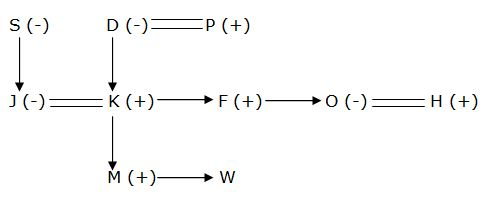# SBI PO 2020 Reasoning Ability Questions 2020 (Day-02)

Dear Aspirants, Our IBPS Guide team is providing new series of Reasoning Questions for SBI PO 2020 so the aspirants can practice it on a daily basis. These questions are framed by our skilled experts after understanding your needs thoroughly. Aspirants can practice these new series questions daily to familiarize with the exact exam pattern and make your preparation effective.

[WpProQuiz 7583]

Seating

Directions (1-5): Study the following information carefully and answer the below questions.

Eight persons M, N, O, P, Q, R, S and T are sitting in a row. Four persons are facing north and another four are facing south, but not necessarily in the same order.

O sits third to the right of S. P sits third to the right of R. P sits third to the right of Q. N sits third to the left of S. M sits third to the left of T. S sits third to the left of O. S sits third to the left of N. O sits at the one of the extreme ends. N is not an immediate neighbour of M. M is not facing north direction. N is not an immediate neighbour of R.  R sits third to the left of P.

1) Which among the following pairs sit at the extreme end of the row?

a) O and R

b) N and O

c) Q and O

d) O and T

e) None of these

2) Who among the following persons sits third to the right of M?

a) No one

b) T

c) P

d) N

e) O

3) Four of the five are alike in a certain way thus form a group. Find the one which does not belongs to the group?

a) P

b) R

c) T

d) S

e) N

4) If all the persons are arranged in alphabetical order from right to left, then how many persons position will get unchanged?

a) One

b) Two

c) Three

d) No one

e) None of these

5) Who among the following persons sits fourth to the left of T?

a) No one

b) O

c) M

d) R

e) S

Inequality

Directions (6-8): In each question, relationship between different elements is shown in the statements. The statements are followed by conclusions. Study the conclusions based on the given statements and select the appropriate answer.

6) Statements:

A < D ≤ R < T = O;             M ≥ S > R < P;           M ≤ U < V

Conclusions:

I) D < V

II) T > M

III) M ≥ O

IV) U ≥ O

a) Only I follow

b) Only II and III follow

c) Either II or III follow

d) Either II or III and I follow

e) None of these

7) Statements:

B ≥ Y < R = O > S;     Z ≤ A < Y > T;   C ≤ E = T < F

Conclusions:

I) O > Z

II) Y < C

III) S < A

IV) T < O

a) Only I follow

b) Only II and III follow

c) Only I and IV follow

d) All I, II and IV follows

e) None follows

8) Statements:

S < M > A = R ≥ I;             P > I ≤ N < H;            L ≤ H = O > V

Conclusions:

I) M > H

II) O ≥ M

III) A > O

IV) H ≤ M

a) None follows

b) Only II follows

c) Either I or II follows

d) Only IV follows

e) Either I or IV follows

Blood relation

Directions (9-10): Study the following information carefully and answer the below questions.

In a family containing 10 members; F and K are siblings. J is the Daughter of S who is the mother-in-law of K. O is the only daughter of P. M is the Nephew of O who is married to H. D is the paternal grandmother of M who is the brother of W. F is the brother of O and he is unmarried. D has only three children.

9) How is P related to W?

a) Father

b) Brother

c) Cousin

d) Uncle

e) Grand father

10) How is F related to J?

a) Sister-in-law

b) Brother

c) Spouse

d) Brother-in-law

e) None of these

Directions (1-5):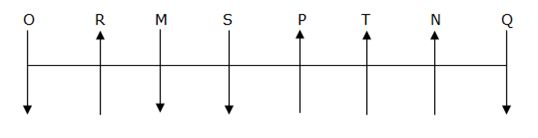O sits third to the right of S. S sits third to the left of O. N sits third to the left of S.

S sits third to the left of N.

Case1: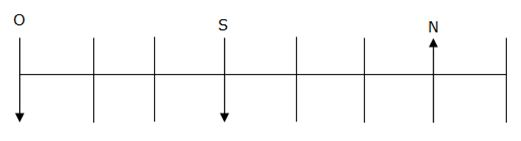Case2: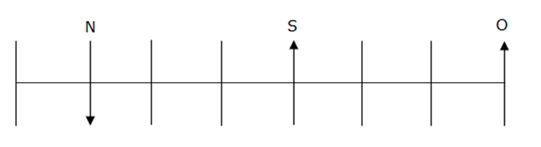P sits third to the right of R. P sits third to the right of Q. R sits third to the left of P. N is not an immediate neighbour of R.

Case1: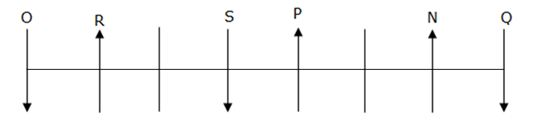Case2: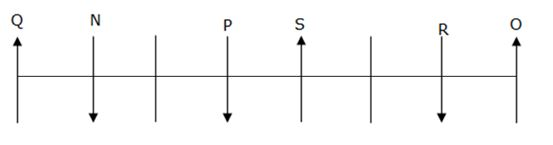M sits third to the left of T. M is not facing north direction. N is not an immediate neighbour of M.

Case2: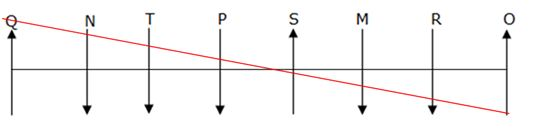Case1: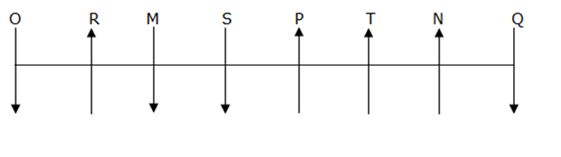Directions (6-8):

I) D < V (D ≤ R < S ≤ M ≤ U < V)–> True

II) T > M (M ≥ S > R < T)–> False

III) M ≥ O          (M ≥ S > R < T = O)–> False

IV) U ≥ O (U ≥ M ≥ S > R < T = O)–> False

So, Either II or III and I follow

I) O > Z (Z ≤ A < Y < R = O)–> True

II) Y < C (C ≤ E = T < Y)–> False

III) S < A           (A < Y < R = O > S)–> False

IV) T < O (T < Y < R = O)–> True

So, Only I and IV follow

I) M > H (M > A = R ≥ I ≤ N < H)–> False

II) O ≥ M (M > A = R ≥ I ≤ N < H = O)–> False

III) A > O          (A = R ≥ I ≤ N < H = O)–> False

IV) H ≤ M (M > A = R ≥ I ≤ N < H)–> False

So, none follows

Directions (9-10):